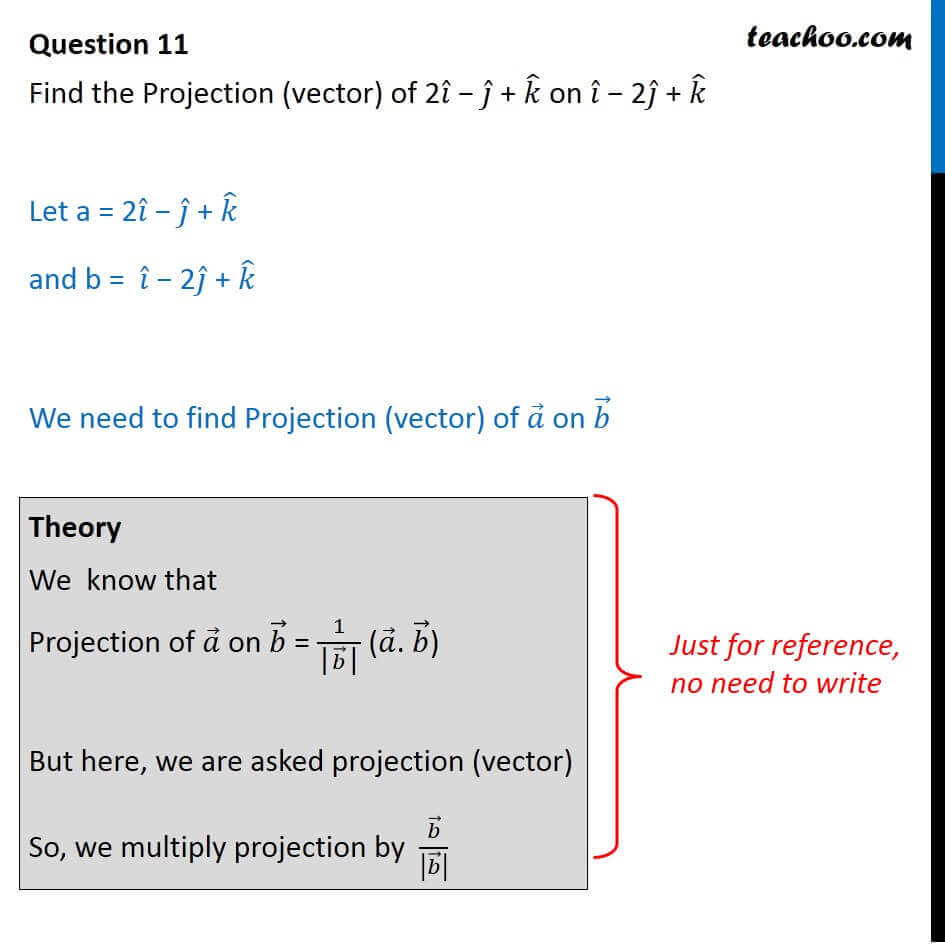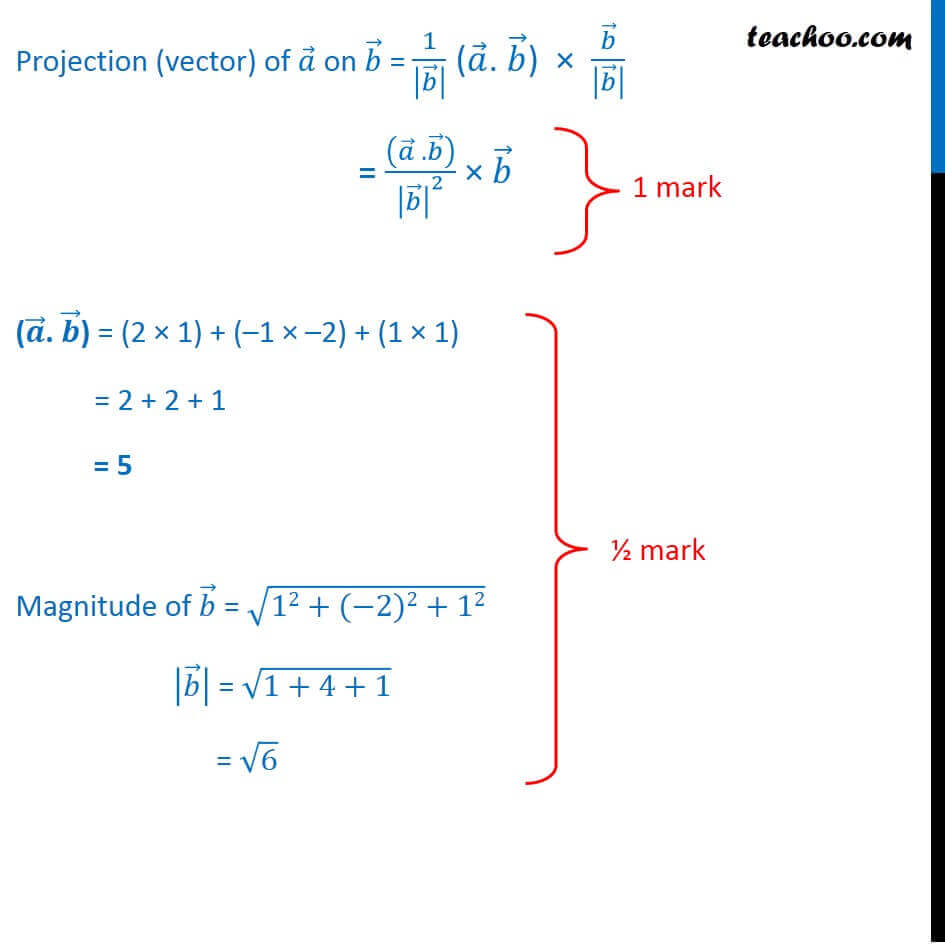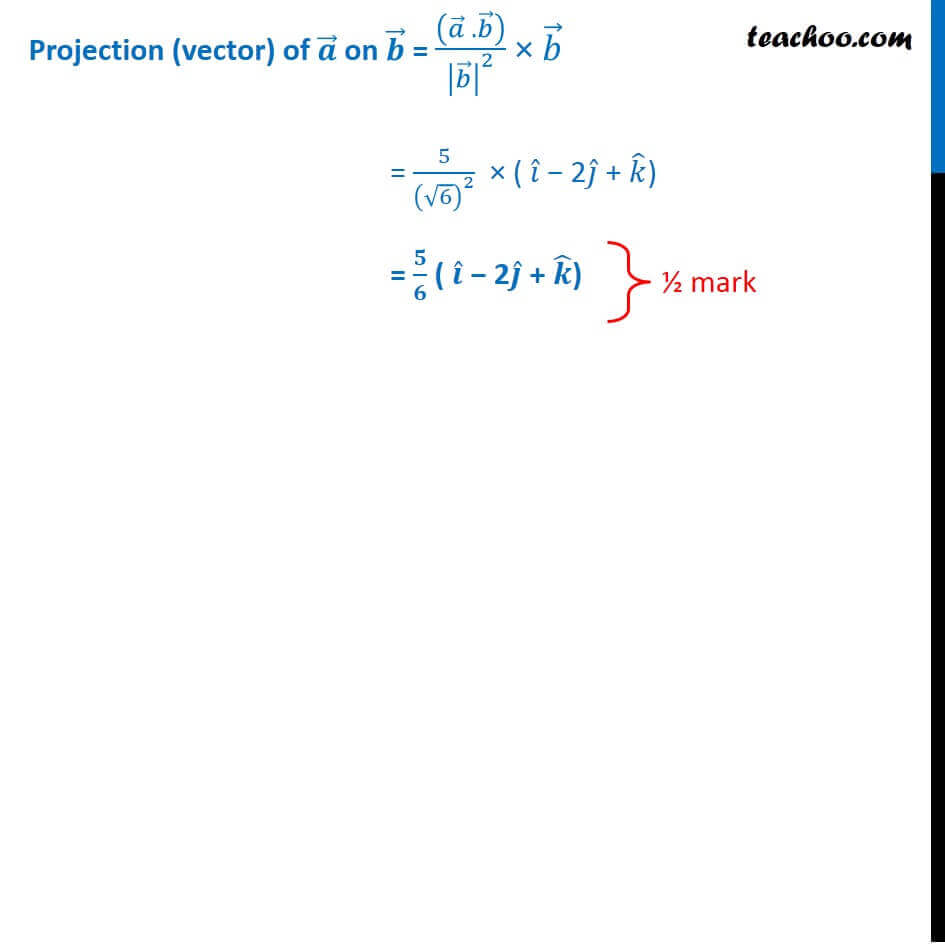CBSE Class 12 Sample Paper for 2018 Boards

Class 12
Solutions of Sample Papers and Past Year Papers - for Class 12 Boards

### Find the Projection (vector) of 2i − j + k on i − 2j + k

This is a question of CBSE Sample Paper - Class 12 - 2017/18.Get live Maths 1-on-1 Classs - Class 6 to 12

### Transcript

Question 11 Find the Projection (vector) of 2𝑖 ̂ − 𝑗 ̂ + 𝑘 ̂ on 𝑖 ̂ − 2𝑗 ̂ + 𝑘 ̂ Let a = 2𝑖 ̂ − 𝑗 ̂ + 𝑘 ̂ and b = 𝑖 ̂ − 2𝑗 ̂ + 𝑘 ̂ We need to find Projection (vector) of 𝑎 ⃗ on 𝑏 ⃗ Theory We know that Projection of 𝑎 ⃗ on 𝑏 ⃗ = 1/("|" 𝑏 ⃗"|" ) (𝑎 ⃗. 𝑏 ⃗) But here, we are asked projection (vector) So, we multiply projection by 𝑏 ⃗/|𝑏 ⃗ | Projection (vector) of 𝑎 ⃗ on 𝑏 ⃗ = 1/|𝑏 ⃗ | (𝑎 ⃗. 𝑏 ⃗) × 𝑏 ⃗/|𝑏 ⃗ | = ((𝑎 ⃗ . 𝑏 ⃗ ))/|𝑏 ⃗ |^2 × 𝑏 ⃗ (𝒂 ⃗. 𝒃 ⃗) = (2 × 1) + (–1 × –2) + (1 × 1) = 2 + 2 + 1 = 5 Magnitude of 𝑏 ⃗ = √(12+(−2)2+12) |𝑏 ⃗ | = √(1+4+1) = √6 Projection (vector) of 𝒂 ⃗ on 𝒃 ⃗ = ((𝑎 ⃗ . 𝑏 ⃗ ))/|𝑏 ⃗ |^2 × 𝑏 ⃗ = 5/(√6)^2 × ( 𝑖 ̂ − 2𝑗 ̂ + 𝑘 ̂) = 𝟓/𝟔 ( 𝒊 ̂ − 2𝒋 ̂ + 𝒌 ̂)